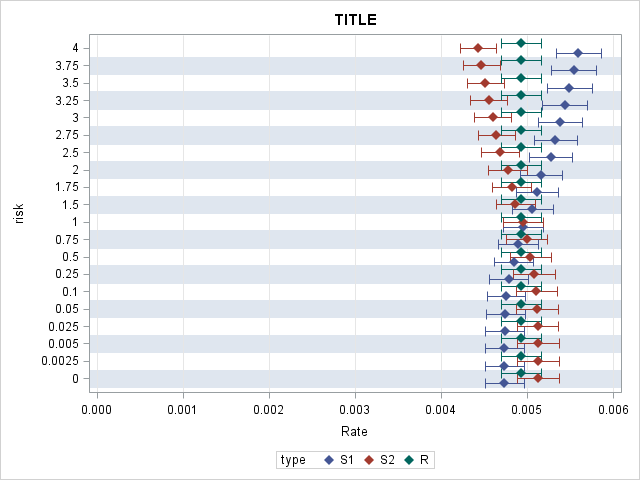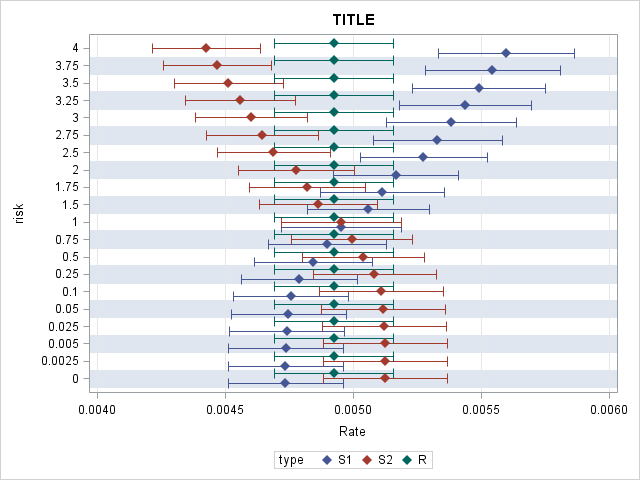## proc sgplot / custom scale for xaxis

I'd like to create a Plot 1 without the empty space with no information. Ideally, I want to show xaxis only for the range of 0.004-0.006 keeping CI95% brackets as narrow as shown in plot1. However, every time I use min, max, offsetmin, offsetmax options or values=from to by, or xaxis min=0.004 options in the code, 95%CI brackets become so wide as shown in Plot 2 which is not desirable. Please help how to create Plot 1 but without the empty space in front.

Plot 1. Desirable dimension and style of plot for narrow CI95%. But I would like to eliminate the space without information until from 0 to 0.004Plot 2. Undesirable style but resulted from code with xaxis min=0.004k

``````proc sgplot data=data;
scatter y=Risk x=Rate/ xerrorlower=LowerCL xerrorupper=UpperCL
markerattrs=(symbol=diamondfilled)
group=Type groupdisplay=cluster;
xaxis grid min=0;
yaxis grid colorbands=odd discreteorder=data type=discrete;
label Rate = "Rate";
run;``````

1 ACCEPTED SOLUTION

Accepted SolutionsJay54
Meteorite | Level 14

## Re: proc sgplot / custom scale for xaxis

The CI bar widths are scaled to the x-axis based on the values you provide in the LowerCL and UpperCL variables in the data set.  It is the same in both the graphs.  Plot 1 has bigger range on the x-axis, so the same bar widths look smaller.  The only way to change them is if you change the values in the LowerCL and UpperCL variables in the data set.

5 REPLIES 5Jay54
Meteorite | Level 14

## Re: proc sgplot / custom scale for xaxis

Don't set the min=0 on the xaxis statement.  Then you will not get the empty space.

The CI bar widths depend on the values in the data you are providing and scaled with the x-axis.

## Re: proc sgplot / custom scale for xaxis

@Jay54

Thanks Sanjay. Commenting out min=0 resulted in widened 95CI. Desirable plot is narrow and tall CI95 as shown in plot 1.

``````proc sgplot data=p.alb_conc;
scatter y=Risk x=Rate/ xerrorlower=LowerCL xerrorupper=UpperCL
markerattrs=(symbol=diamondfilled)
group=Type groupdisplay=cluster;
/*   xaxis grid min=0.004;*/
yaxis grid colorbands=odd discreteorder=data type=discrete;
label Rate = "Rate";
title 'hi';
run;``````Jay54
Meteorite | Level 14

## Re: proc sgplot / custom scale for xaxis

The CI bar widths are scaled to the x-axis based on the values you provide in the LowerCL and UpperCL variables in the data set.  It is the same in both the graphs.  Plot 1 has bigger range on the x-axis, so the same bar widths look smaller.  The only way to change them is if you change the values in the LowerCL and UpperCL variables in the data set.

## Re: proc sgplot / custom scale for xaxis

Sanjay, I'm using noautolegend, do you know how to label for each type=discrete in legend on the side of the plot? any reference document for the similar plot?Jay54
Meteorite | Level 14

## Re: proc sgplot / custom scale for xaxis

If you leave out the NOAUTOLEGEND you will get the default legend at the bottom.  You can customize its position by using a KEYLEGEND / position=right across=1;

Discussion stats
• 5 replies
• 1478 views
• 2 likes
• 2 in conversation